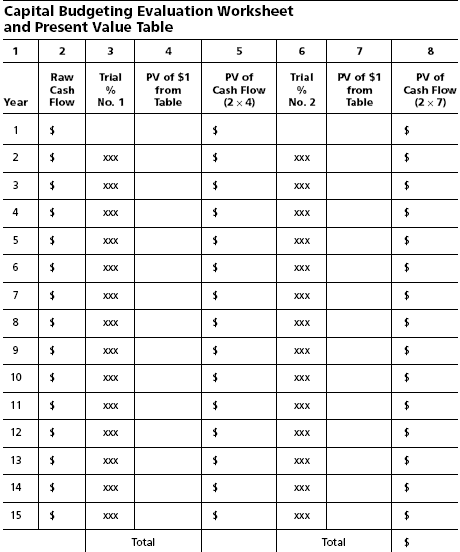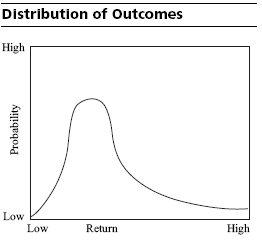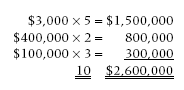Connect with us

# Capital Budgeting Evaluation Worksheet – Improving EstimatesPublished

on

Once the cash flows have been determined from the capital budgeting cash flow worksheet, they are listed on the capital budgeting evaluation worksheet. To be able to fill the worksheet, you will need present value table for some periods (e.g.: 5-15 years), at various rates (e.g.: from 5 percent to 30 percent). It is best to keep this table together with the capital budgeting worksheet so that later referral to the worksheet will not result in questions concerning the origin of the numbers used in your calculation.The use of the evaluation worksheet is straightforward: The cash flows are taken from the cash flow worksheet and are listed in column 2. In column 3, the first trial percentage rate is listed to generate the present value of income flow. Column 4 is read directly from the present value table for the first trial interest rate. Those numbers are filled into the form from the matrix. Column 5 is the multiplication of the cash flow from column 2 by the present value factor from column 4. Column 6 is used for a second trial percentage. Once again the process is repeated and the present value rates are included in column 7 for the second percentage selected. Column 8 is again calculated by the multiplication of the cash flows from column 2 and, this time, the present value numbers in column 7. In this manner, two trials can be made to evaluate the present values of a single cash flow estimate over two different discount rates. Using these worksheets, the cash flows for various proposals may be compared.

Improving the Estimates

In most cases, the unfortunate truth is that things normally can get only a little bit better but a whole lot worse than expected. Therefore, if the distribution of possible outcomes is considered, it probably would be skewed to the left, in that there is a greater number of unfortunate outcomes than fortunate ones. The possibility of improvement is also limited by the production capabilities. Therefore, the limiting factor on the right side may be plant capacity. Since capacity is normally added in significant increments as opposed to one or two units at a time, there is no continuum of outcome possibilities. Instead, production capacities occur in steps. Without getting into the problem of analyzing additional production quantities, consider the problem of improving the estimates from the standpoint of fixed or limited capacity.The problem encountered in capital budgeting, as in all other planning, is that a “most likely” figure is normally offered. However, other alternatives should be considered. This situation is not uncommon: “most likely” sales estimate is \$300,000; “best case” (limited by capacity) is \$400,000; “worst case” sales estimate is \$100,000. One way to use this information is to multiply each by some estimated probability. For example, the probable outcome for “most likely” may be estimated as 5 chances out of 10; “best” is 2 chances out of 10; and “worst” is 3 chances out of 10. To calculate the expected outcome, we start by multiplying the “most likely” sales estimate by 5 and repeat this process for each outcome.

So:Sum up the probabilities of 5, 3, and 2 for a total of 10. Finally, divide the sum of the multiplications by the sum of the probabilities.

The expected value is: \$2,600,000/10 = \$260,000. The resulting expected value amount of \$260,000 is less than the most likely figure of \$300,000 and reflects the fact that the curve is skewed to the left. While \$300,000 is still most likely, a conservative estimate of \$260,000 is also reasonable.

Although not impressive statistically, this approach does make use of more information; this fact usually would justify its inclusion in cash flow projections. Understand that each of the figures—the sales figures and the probabilities associated with each of the three cases—is an estimate. In making these estimates, you should take care to ask a lot of what-if questions.

When trying to evaluate what is behind the numbers, it is also extremely important to evaluate the information sources. As mentioned previously, engineers may tend to underestimate time to complete projects and thereby underestimate costs. Marketing and sales personnel may overestimate sales and sales potentials. Then, ask:

How good are the forecasts of the market, the economic conditions and the expectation of future cash flows?

Often it is necessary to question where the numbers came from, who generated them, on what assumptions they were based, and what data were used. It is helpful to know the sources of data, the age of the data, and the method of generation.

Often these sources are used:

1. Government publications, which give useful information on the trends in the economy, consumer spending, and other market information
2. Private company publications such as Chase Econometric, Dow Jones, and the like.
4. Newspapers

Experience in the industry usually helps provide an understanding about the availability and reliability of certain information and data sources.

A major task in capital budgeting is estimating future cash flows. The quality of the final budget and plan is really only as good as the accuracy of the estimates. You should have efficient procedures set up to collect the information necessary for capital budgeting decisions. Try to standardize this information as much as possible for all investment proposals; otherwise, proposals cannot be compared objectively.

One of the more difficult capital budgeting problems to evaluate concerns projects associated with environmental protection or safety. It is difficult in those projects to quantify the net cash flows because in most cases the benefit to you is more in the nature of a cost avoidance.

The reason the expected benefits from a particular project are expressed in terms of cash flows rather than in terms of income is that cash is central to all your decisions. You invest cash now in the hopes of receiving cash returns of a greater amount in the future. Only cash receipts can be reinvested or paid to stockholders in the form of dividends. Thus cash, not income, is what is important in capital budgeting.

Capital Budgeting

Capital Budgeting Model: Risk-Return Relationship, Cost Of Capital Calculation, Capital Budgeting Worksheet

Capital Budget Evaluation – Cash Flow and Capital Budget Proposal

Comparison of the Internal Rate of Return (IRR) and Net Present Value (NPV) Method

Are you looking for easy accounting tutorial? Established since 2007, Accounting-Financial-Tax.com hosts more than 1300 articles (still growing), and has helped millions accounting student, teacher, junior accountants and small business owners, worldwide.